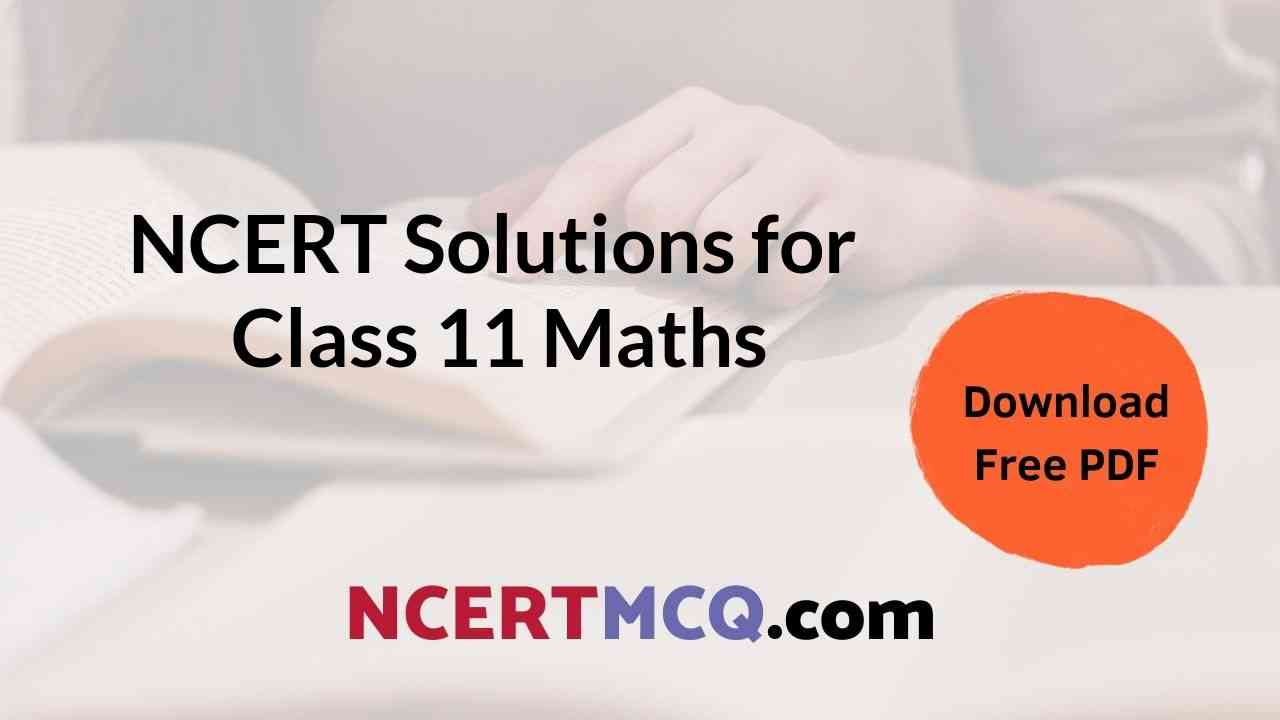## Class 11th Maths NCERT Solutions | NCERT Solutions of Maths Class 11

Maths NCERT Solutions Class 11 | Maths Class 11 NCERT Solutions

Maths 11 NCERT Solutions Chapter 1 Sets

NCERT Class 11th Maths Solution Chapter 2 Relations and Functions

NCERT Solutions Class 11th Maths Chapter 3 Trigonometric Functions

NCERT Maths Book Class 11 Solutions Pdf Chapter 4 Principle of Mathematical Induction

Solution of Maths Class 11 Chapter 5 Complex Numbers and Quadratic Equations

Class 11 Maths NCERT Book Solutions Chapter 6 Linear Inequalities

Class 11 Math NCERT Solution Chapter 7 Permutations and Combinations

NCERT Maths Solutions Class 11 Chapter 8 Binomial Theorem

NCERT 11 Maths Solutions Chapter 9 Sequences and Series

CBSE Class 11 Maths Solutions Chapter 10 Straight Lines

Class 11 NCERT Maths Solutions Chapter 11 Conic Sections

NCERT Solutions of Maths Class 11 Chapter 12 Introduction to three Dimensional Geometry

Maths NCERT Solutions Class 11 Chapter 13 Limits and Derivatives

Class 11 Maths Solutions Chapter 14 Mathematical Reasoning

NCERT Maths Class 11 Solutions Chapter 15 Statistics

NCERT Solutions of Class 11th Maths Chapter 16 Probability